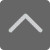• 上海

# 小学口算题大全：二年级100以内加减法口算题（四）

2018-11-02 16:33:39 　来源：网络 文章作者：网络

## — — 学而思小学课程在线预约 — —点击预约→免费的1对1学科诊断及课程规划

94-56=

12+9+41=

44-18=

63-17=

4+22+8=

15+63=

42-27=

33+2+38=

49+39=

71+16=

77+21+9=

97-83=

71-59=

82+7+3=

76-65=

63-3=

62+5+15=

51+15=

54+12=

80+8+2=

73-52=

69+17=

59+30+10=

53+17=

63-7=加入QQ群，与更多家长交流经验！！！
 上海小学交流群：639215153 上海初中交流群：611612914 上海高中交流群：959031473 上海幼升小交流群：772707735

### 预约课程更多 >